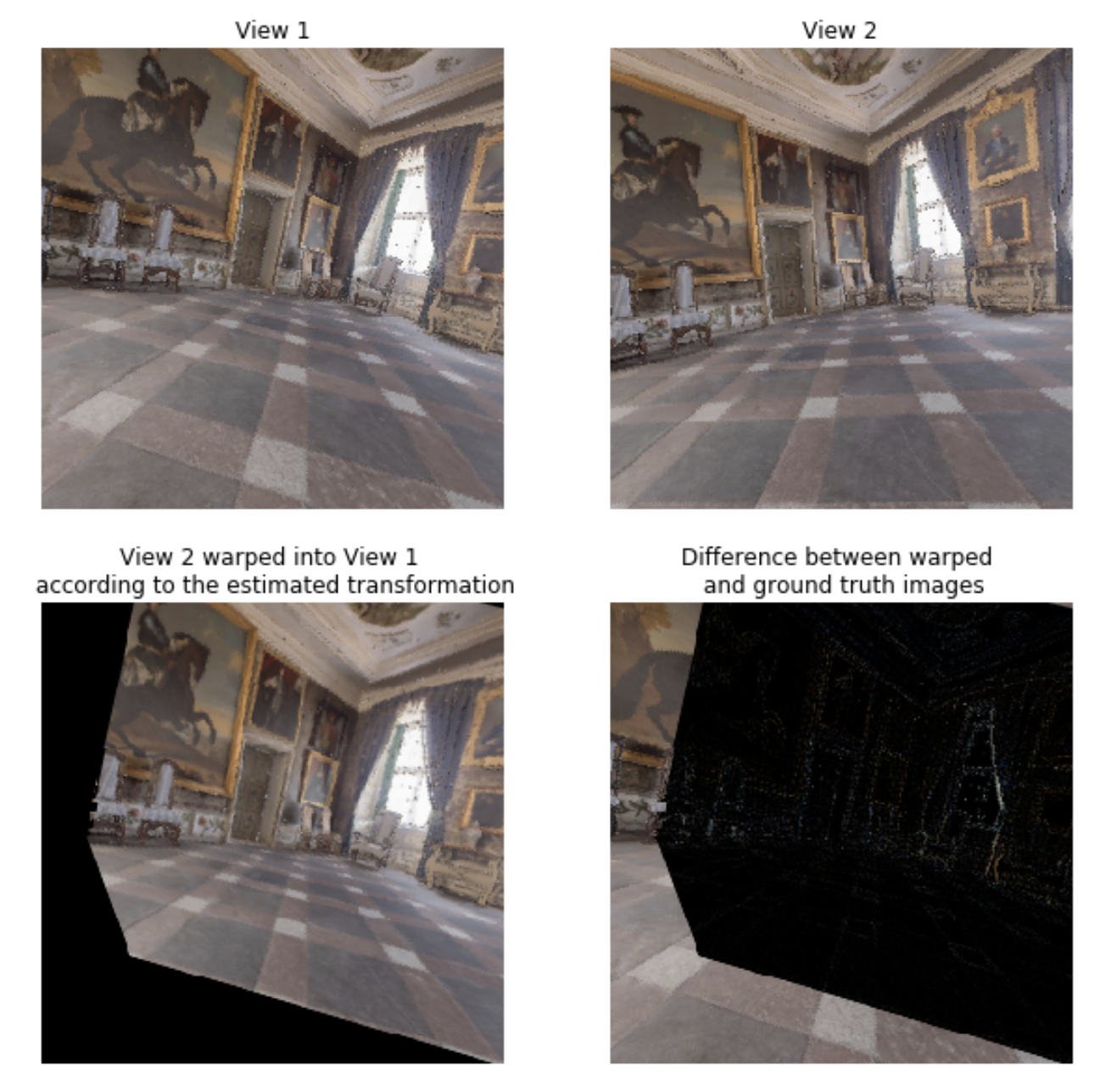# View, Transform and Warp

Demonstrates how to extract camera parameters in the scene and how these camera parameters relate to the given views. We create 2 cameras and use RGB and Depth information to construct transformation from a view of camera 1 to camera 2 and validate that transformation comparing projected and original views.

## Randomly permute the rotation

```def uniform_quat(original_angle):
original_euler = quaternion.as_euler_angles(original_angle)
euler_angles = np.array([(np.random.rand() - 0.5) * np.pi / 9. + original_euler,
(np.random.rand() - 0.5) * np.pi / 9. + original_euler,
(np.random.rand() - 0.5) * np.pi / 9. + original_euler])
quaternions = quaternion.from_euler_angles(euler_angles)

return quaternions```

## Generate two random, overlapping views

```depths = []
rgbs = []
cameras = []

for i in range(0, 2):
rotation = uniform_quat(init_rotation)
translation = init_translation + np.random.rand(3,) * 0.5 - 0.25

obs = env._sim.get_observations_at(position=translation, rotation=rotation, keep_agent_at_new_pose=True)
depths += [obs["depth"][...,0]]
rgbs += [obs["rgb"]]

cameras += [env._sim.get_agent_state()]

env.close()```

## Intrinsic parameters, K

```K = np.array([
[1 / np.tan(hfov / 2.), 0., 0., 0.],
[0., 1 / np.tan(hfov / 2.), 0., 0.],
[0., 0.,  1, 0],
[0., 0., 0, 1]])

# Now get an approximation for the true world coordinates -- see if they make sense
# [-1, 1] for x and [1, -1] for y as array indexing is y-down while world is y-up
xs, ys = np.meshgrid(np.linspace(-1,1,W), np.linspace(1,-1,W))
depth = depths.reshape(1,W,W)
xs = xs.reshape(1,W,W)
ys = ys.reshape(1,W,W)

# Unproject
# negate depth as the camera looks along -Z
xys = np.vstack((xs * depth , ys * depth, -depth, np.ones(depth.shape)))
xys = xys.reshape(4, -1)
xy_c0 = np.matmul(np.linalg.inv(K), xys)

# Now load in the cameras, are in the format camera --> world
# Camera 1:
quaternion_0 = cameras.sensor_states['depth'].rotation
translation_0 = cameras.sensor_states['depth'].position
rotation_0 = quaternion.as_rotation_matrix(quaternion_0)
T_world_camera0 = np.eye(4)
T_world_camera0[0:3,0:3] = rotation_0
T_world_camera0[0:3,3] = translation_0

# Camera 2:
translation_1 = cameras.sensor_states['depth'].position
quaternion_1 = cameras.sensor_states['depth'].rotation
rotation_1 = quaternion.as_rotation_matrix(quaternion_1)
T_world_camera1 = np.eye(4)
T_world_camera1[0:3,0:3] =  rotation_1
T_world_camera1[0:3,3] = translation_1

# Invert to get world --> camera
T_camera1_world = np.linalg.inv(T_world_camera1)

# Transformation matrix between views
# Aka the position of camera0 in camera1's coordinate frame
T_camera1_camera0 = np.matmul(T_camera1_world, T_world_camera0)

# Finally transform actual points
xy_c1 = np.matmul(T_camera1_camera0, xy_c0)
xy_newimg = np.matmul(K, xy_c1)

# Normalize by negative depth
xys_newimg = xy_newimg[0:2,:] / -xy_newimg[2:3,:]
# Flip back to y-down to match array indexing
xys_newimg *= -1```

## And visualise this new transformation